|
|### Introduction to the Metrology of VNA Measurement

May 31, 2022

#### Introduction

A Vector Network Analyzer (VNA) makes RF measurements of reflection coefficients. To be very meticulous, each measurement should be accompanied by an uncertainty with a specified statistical confidence interval. A typical reflection measurement might be -32 dB ± 0.5 dB (95% confidence interval). This is to say that the real reflection is between -31.5 and -32.5 dB with 95% (2σ) confidence. There is a 5% chance that the real value is outside of these bounds, but it is usually possible to recalculate the uncertainty for 99%, or 3σ confidence. The real value will lie within a larger window. A full metrologically sound measurement will have all three factors; the value, the uncertainty, and the confidence interval of the uncertainty.

To understand the measurement uncertainty, or accuracy, it is important to understand the factors which contribute to it. A VNA measurement will be affected by both systematic and random errors. The process of user calibration mostly corrects systematic errors but leaves some residual errors. Random factors cannot be corrected by calibration but may be minimized through careful measurement methodology. This paper will describe the types of calibration that might be employed, the errors which these methods correct, the residual errors which remain, the mitigation of random errors, and how to estimate the uncertainty of a measurement taking all of these things into account.

#### What is Metrology?

Metrology is the science of measurement. A valid and repeatable measurement must be expressed in a common unit system and the accuracy of that measurement may be expressed as a statistical uncertainty. It is all very well to deliver a measurement result with 12 digits, but how many of them are meaningful? The science of metrology provides this answer.

The modern system of units is the “SI” system, or Systѐme Internationale, within which seven primary units are defined; the Meter, the Second, the Ampere, the Kelvin, the Mole, the Candela, and the Kilogram. All other units in use are derived from these. All seven primary units may be reproduced by observing certain physical phenomena.For instance, the meter is defined in terms of a certain number of wavelengths of a spectral emission line of Krypton-86 where once it was defined by a single physical metal prototype. At present, the kilogram is defined by a cylindrical artifact composed of a Platinum and Iridium alloy housed in a double bell jar and stored in a vault at the International Bureau of Weights and Measures (BIPM) in Paris. Up until a few years ago, the kilogram was defined by a cylindrical artifact composed of a Platinum and Iridium alloy housed in a double bell jar and stored in a vault at the International Bureau of Weights and Measures (BIPM) in Paris. This was the last remaining primary standard unit defined by a physical artifact.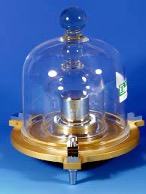Figure 1 – The “IPK”, Kilogram Standard artifact, image courtesy of BIPM, Paris

It is the task of various national standards organizations such as the “National Institute of Standards and Technology” (NIST) in the United States or the Federal Institute of Metrology (METAS) in Switzerland to provide the primary means of measurement verification. Commercial calibration laboratories will have primary standards which must be periodically certified by a national lab and may create or use secondary standards which are verified by comparison to a primary standard. Commercially available calibration kits are an example of a secondary standard. Off-the-shelf secondary standards come in a variety of grades from low, economy grades to precision (and more expensive) grades. The uncertainties of each standard should be available to the user in order to ascertain its particular level of precision.

A good measurement must be traceable. That is, the measurement should be performed by an instrument that is calibrated in such a way that the uncertainty can be traced back to a primary standard that has been certified by a national metrological laboratory. The calibration laboratory at Copper Mountain Technologies is ISO/IEC 17025:2017 certified through the ANSI National Accreditation Board (ANAB) for RF measurement and calibration up to 54 GHz.

#### VNA Measurement

A vector network analyzer (VNA) measures S-parameters. These are expressed as the ratios of voltage waves traveling in either a forward or reverse direction on a conductor or within a waveguide. Where an oscilloscope can only measure the composite voltage signal at a point on a conductor, the VNA can determine the magnitude and phase of two signals, forward and reverse traveling or incident and reflected, which make up that signal. A simplified block diagram of a VNA is shown in Figure 2 below.

If the stimulus is switched to the left-hand, Port 1 side, the R1 receiver measures the magnitude of the voltage wave leaving the VNA. If there is a reflected wave coming back to Port 1, the A receiver would measure it. If the two ports were connected, then receiver B would measure the amount of the R1 signal entering Port 2. If the connection has low loss, then B would essentially equal R1 except for a phase delay due to the length of the connection. The Bridge separates signals by direction into forward and reverse waves.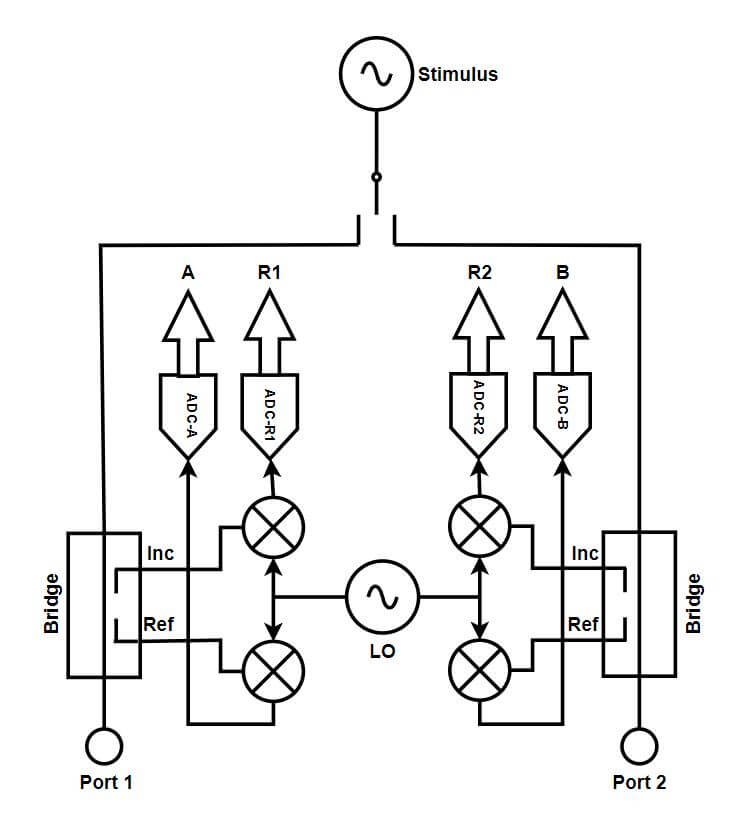Figure 2 – Simplified VNA Block Diagram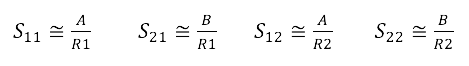The 2-port S-parameters are derived from the ratio of receiver measurements.

The actual magnitude of the stimulus is not important since it is always measured by a receiver (R). To arrive at the actual S-parameters, calibration is required.

S-parameters are useful for characterizing circuits and antennas. Since most RF circuits are designed for use in 50 or 75 Ohm systems, being able to make measurements directly within those characteristic impedances is extremely useful and most VNAs are designed to operate at one of those two impedances.

S-parameter measurements can determine how much of a signal is reflected from a Device Under Test (DUT) and how much is passing through it. A complex-valued reflection coefficient typically designated with a capital gamma symbol,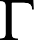, is often used to indicate the magnitude and phase of a reflected signal. The complex impedance encountered by the incident wave determines this reflection: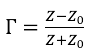Equation 1

Z is the complex impedance seen by the traveling wave and Z0 is the characteristic impedance of the measurement system which will be 50 Ohms in all subsequent discussions. If the incident wave which was launched by a 50 Ohm source sees a 50 Ohm impedance, then the numerator of Equation 1 is zero and there is no reflection,= 0. If the Load is an Open or Short, then there is complete reflection, and= 1 and -1 respectively. The reflection from an Open is in phase with the forward traveling wave and 180 degrees out of phase from a Short.

A DUT may be characterized by a network diagram depicting the four S-parameters. For a linear DUT, these parameters give a complete characterization of its behavior for any sort of signal excitation and with any source or load impedance.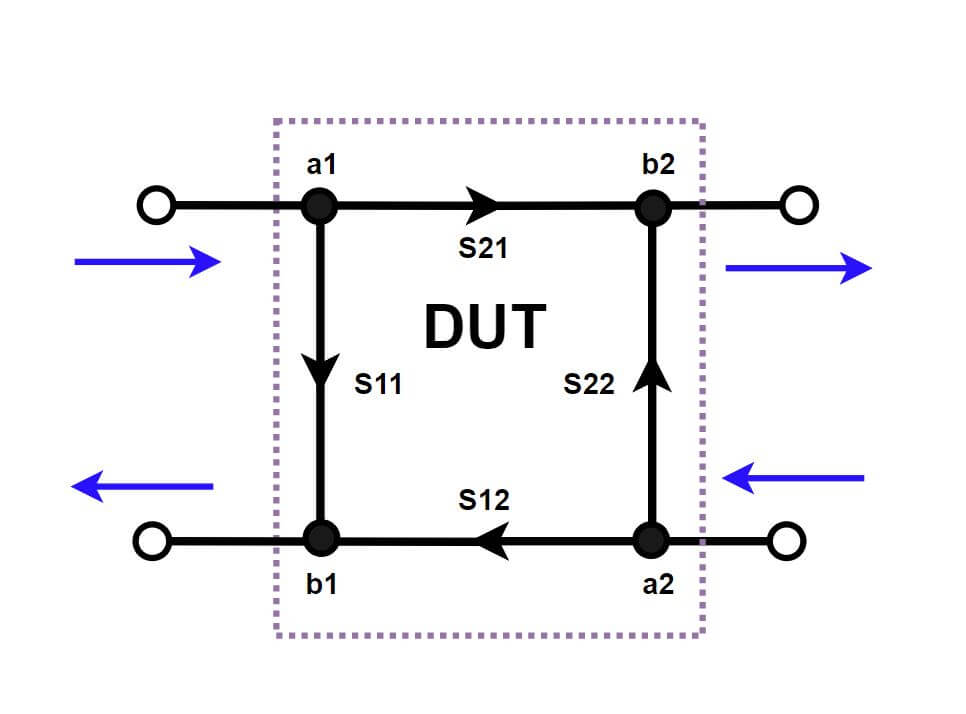Figure 3 – DUT with S-Parameters

Note that the four terminals in the network diagram of Figure 3 do not depict four separate connections to the DUT, there are only two. The different branches are shown in this way to clearly separate voltage waves moving in different directions. In the network above, signals enter and exit the DUT following the direction of the blue arrows.

#### Systematic Errors in VNA Measurements

There are two kinds of VNA measurement errors, random and systematic. Random errors include fluctuations due to receiver noise, mechanical errors, such as connector repeatability or cable flexure, and measurement drift due to environmental influences, such as ambient temperature. For the most part, nothing can be done to prevent these errors completely, but they can be minimized. The temperature control of the measurement environment might be improved, and cable movement reduced by taping cables to the benchtop, for instance. These errors must be recognized and accounted for in a full reckoning of measurement uncertainty.

Systematic errors are built-in and invariant in the measurement system. These errors can be determined by the measurement of known reflection artifacts and then mostly removed from subsequent measurements mathematically. It is never possible to completely remove all errors. Those which remain are called residual errors.

#### 12-Term Error Model

The 12-Term error model is the most common method of systematic error modeling. For a 2-port VNA making measurements in each direction, there are six error terms in one direction and six in the reverse. For brevity, only one direction and six terms will be considered here, as the other six are merely a mirror image. Figure 4 shows the forward error model and Figure 5 the reverse error model.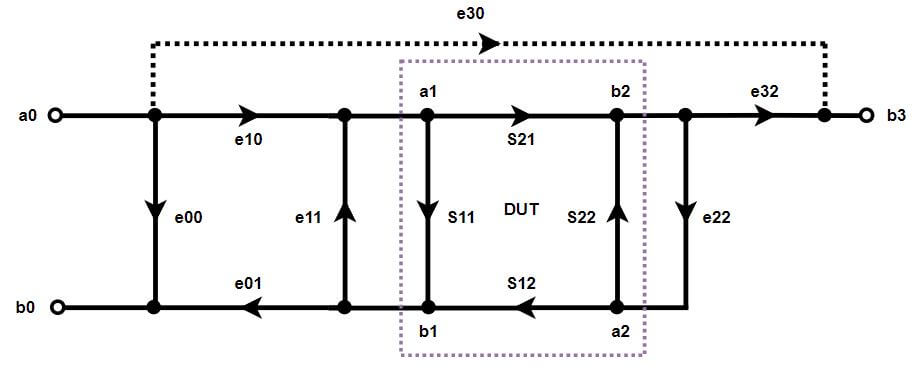Figure 4 – 12-Term Error Model, Forward Direction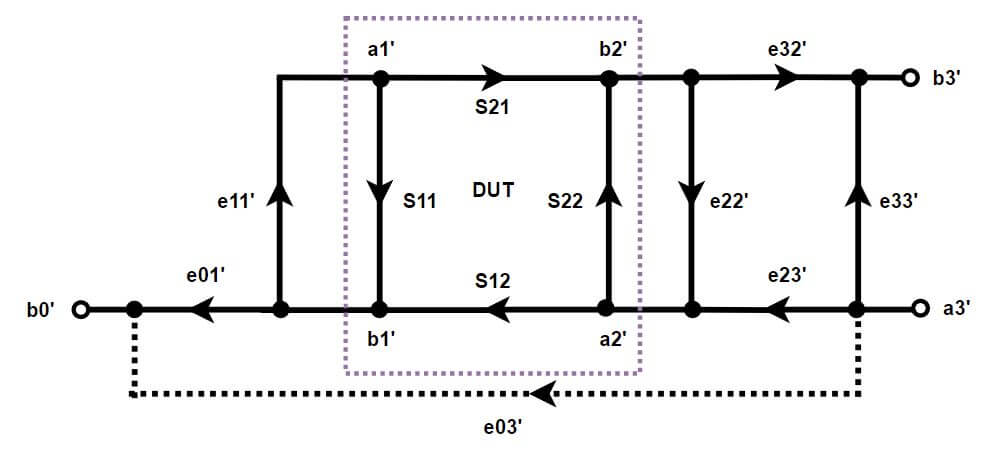Figure 5 – 12 Term Error Model, Reverse Direction

The 14 error contributions form 12 designated errors in the Forward and Reverse directions.

e00, e33’                Directivity Error

e10e01, e23’e32    Reflection Tracking Error

e11, e22                 Source Match Error

e10e32, e23’e01    Transmission Tracking Error

e30, e03                Isolation Error

The Directivity Error, e00, is due to the leakage in the VNA directional bridge. The two ports on the bridge should produce two pure signals. One, a sample of the incident signal leaving the port, and two, a sample of the reflected signal entering the port. In practice, there is some leakage of the incident signal into the reflection port.

Reflection Tracking, e10e01 accounts for the indignities suffered by an incident signal leaving the port, passing through cables and connectors, e10, and being reflected by the DUT, to go through them once again, e01.

Source Match Error, e11, accounts for the complex error in the source impedance of the incident signal as it appears at the input of the DUT. Even if the source impedance of the stimulus of the VNA is a perfect 50 Ohms, small variations in the characteristic impedance of cables and connectors will alter it somewhat.

Transmission Tracking, e10e32, is similar to Reflection Tracking and includes the first part of it, e10, but also includes the errors in the path to the other VNA port, e32.

Load Match Error, e22, is the load impedance error from 50 Ohms as seen at the output of the DUT and includes impedance variations caused by the output cable and connectors and any small load impedance error through the bridge of the VNA itself.

Isolation Error, e30, accounts for any signal which bypasses the DUT entirely. Either through leakage within the VNA itself, which is uncommon, or from the electromagnetic coupling between the two DUT connections, such as that experienced between the probes within a probe station measurement.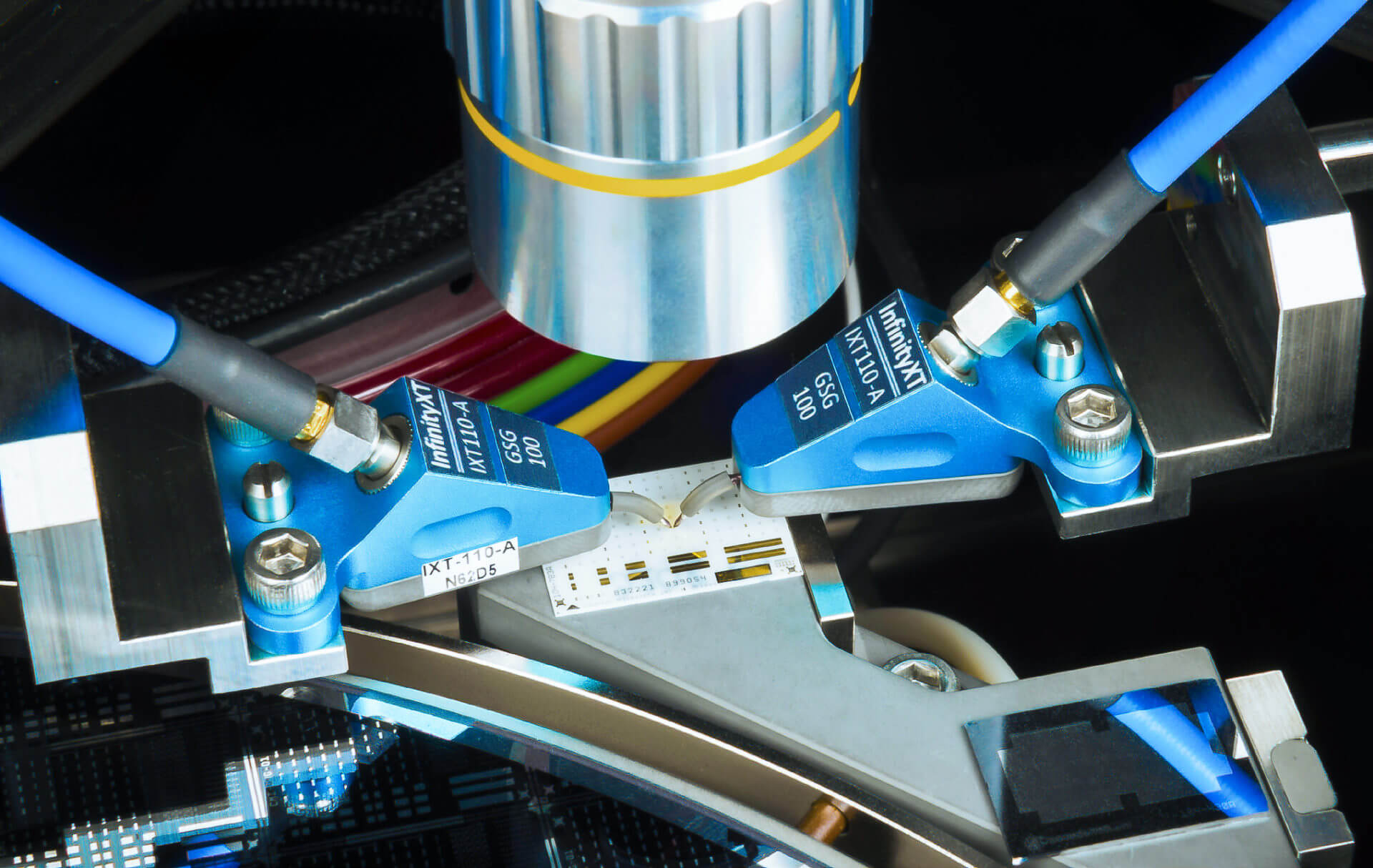Figure 6 – FormFactor coaxial InfinityXT probes measuring ISS calibration standards

Normally the Isolation Error is lower than the receiver noise at even the lowest IF bandwidths and can be safely ignored.

The 12-Term error model is useful as each term represents a real physical source of error and is therefore appealing. Other models, such as the 8-Term model, might also be used, but a better understanding of calibration and residual errors is best based on the more intuitive 12-Term model.

#### Calibration

The process of calibration involves the measurement of several known reflection artifacts and one or more Thru lines. Short-Open-Load-Thru (SOLT) calibration uses a Short, an Open, a 50 Ohm Load, and a Thru standard. The Short and Open are usually characterized by a delay and a third-order polynomial which determines the parasitic capacitance of the Open and parasitic inductance of the Short as a function of frequency. The Load is often assumed to be a perfect 50 Ohms. The delay of the Thru is also given.

Alternatively, all four standards might be defined by a database of reflection vs frequency as measured by a very precisely calibrated VNA. The database method is much more accurate than polynomial characterization.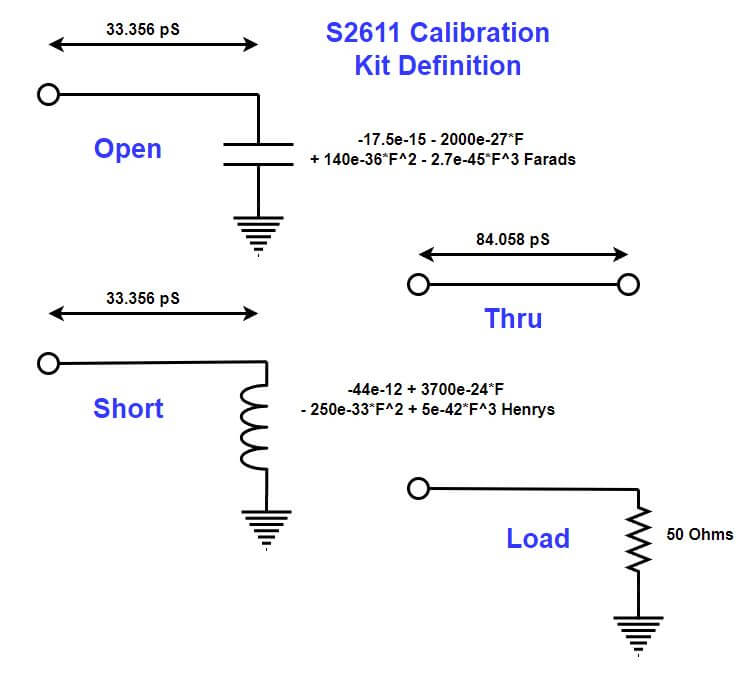Figure 7 – S2611 Calibration Kit Definition

The third-order polynomials and delays are the same for all calibration kits of a certain model. The manufacturer relies on precision manufacturing and machining to enable this, but there will always be some error. Clearly, creating a custom database for each kit is much more accurate but results in a much higher cost.

The kit definition, whether polynomial or databased, is entered in the kit definition table of the VNA.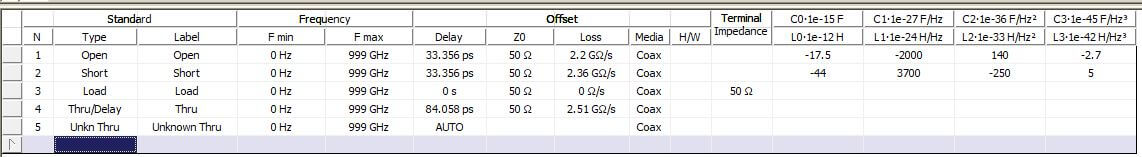Figure 8 – Kit Definition Table

Using the delays and polynomial information from the table, the VNA calculates the actual reflection coefficient of each of the calibration artifacts. The Open will appear as an arc starting at 1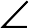0 on the Smith chart (Polar Format) and moves clockwise with frequency as shown below.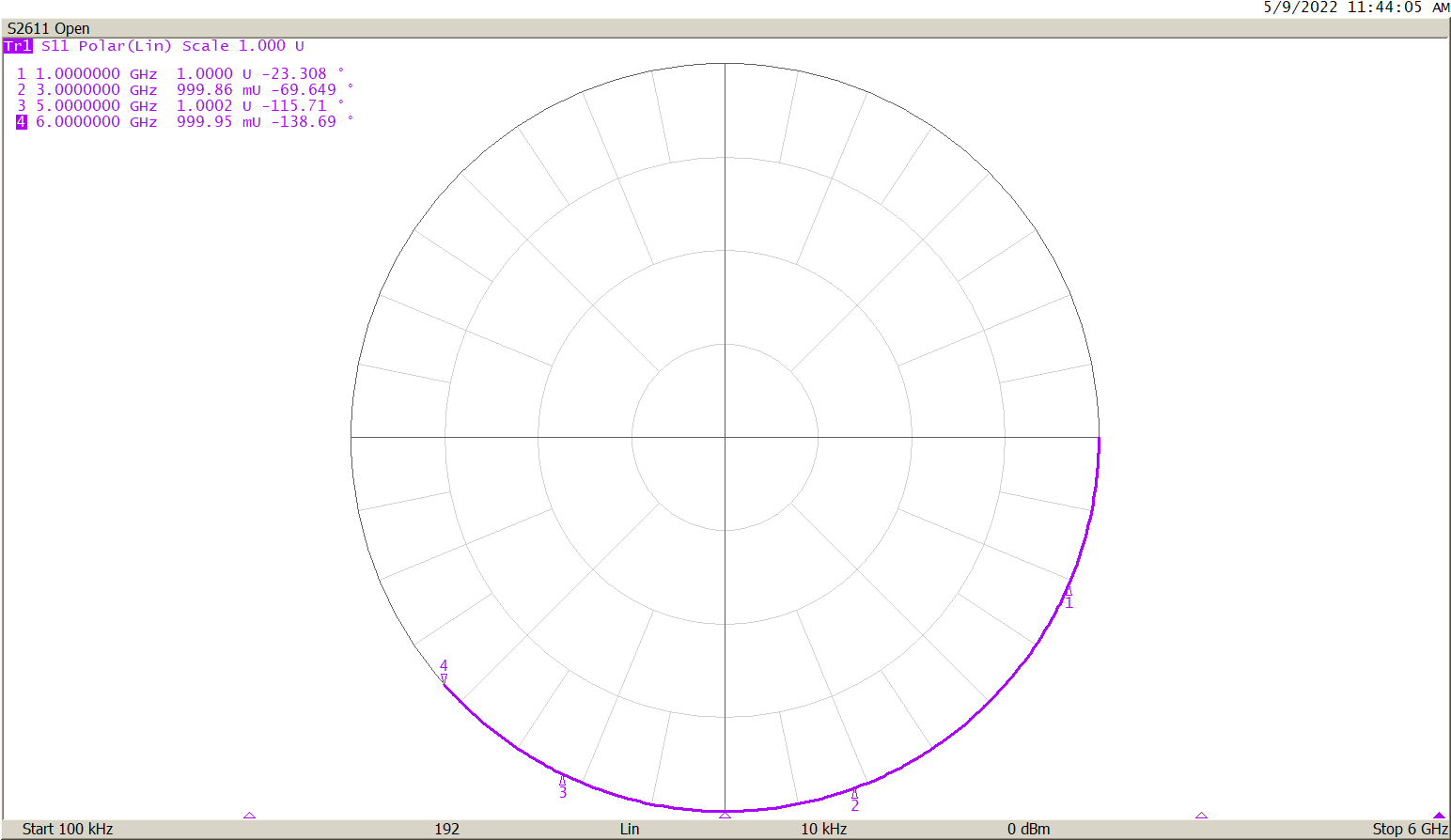Figure 9 – S2611 Calibration Kit Open

The Open is not a dot at 10 because of the 33.356 pS delay between the 3.5 mm Connector and the actual internal Open shown in Figure 7, plus the added effect of the parasitic capacity.

The Short appears as an arc starting at -10 and moves clockwise with frequency.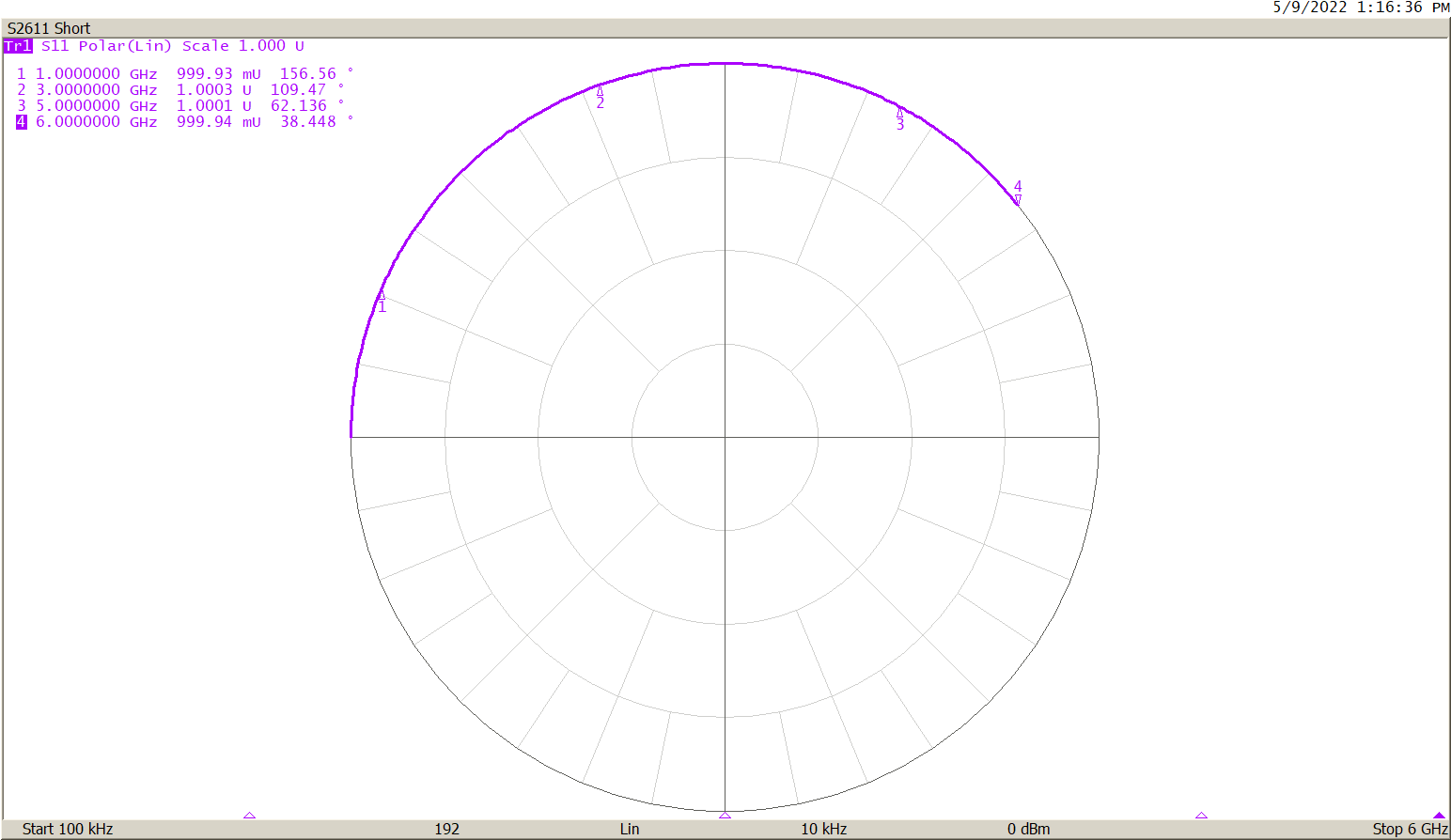Figure 10 – S2611 Calibration Kit Short

For similar reasons, the Short does not appear as a dot at -1 Ð 0.

It should be noted that these arc-like charts are what the Short and the Open actually look like when they are measured. Measuring the Open or the Short after calibration will show these very same results. In fact, even if a calibration artifact is slightly broken in some way, the VNA will display these same results. The process of calibration forces the VNA to match the measurement of the calibration kit Open, Short, and Load to the data provided in the kit definition chart even if damaged.

If the Load is entered in the kit definition as a perfect 50 Ohms, then the measurement of that same Load after calibration will be an extremely low value of reflection. This is somewhat erroneous since an off-the-shelf secondary standard calibration Load usually has a Return Loss no better than 40 dB or so.

A sliding Load is a Load on the end of a variable 50 Ohm air-line. Several measurements of the Load are made at different length offsets, thus rotating the small reflection around the center of the Smith Chart. Averaging these reflections results in a very good estimate of a zero-reflection Load. The uncertainty is much better than a fixed load but a sliding load is quite expensive and very fragile and should be used with great care.

An Automatic Calibration Module (ACM) might be used instead of a mechanical calibration kit. The ACM contains the Open, Short, Load, and Thru artifacts which are electronically switched into place. Each artifact is databased and prior to initiating calibration, this data is uploaded into the VNA. Instead of making four connections to each test cable of the VNA, as is necessary for a mechanical kit, only a single connection is made. This greatly reduces connector uncertainty. Since there are fewer connections made, there is less cable movement, thereby also reducing this uncertainty. The databased Load in the ACM is also a large improvement over the assumption of perfection in the mechanical kit.

An additional feature of the ACM is the Confidence Check. When selected in the VNA menu, a databased factory measurement of an internal 20 dB attenuator is loaded into the VNA memory trace, and the attenuator is switched into place for live measurement. One can then easily compare the live measurement to the factory measurement and perhaps move cables slightly to determine the success of the calibration and the robustness of the test cables.

#### 1-Port SOL Calibration

2-port calibration starts with the performing of 1-port calibration on each port. It is assumed that the errors shown in Figure 4 must be determined. The 1-port error box is shown below: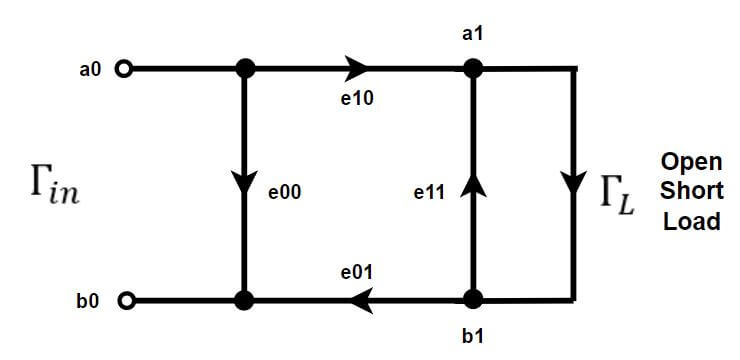Figure 11 – 1-Port Error Box

From Mason’s Rule we have: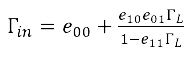Equation 2

If we apply our three known calibration standards, Open, Short, and Load forL, we can solve three equations for three unknowns and arrive at the values for the Reflection Tracking Error, e10e01, the Directivity Error, e00, and the Source Match Error, e11.

#### 2-Port SOLT Calibration

After 1-port calibration has been completed on both ports, the ports are terminated with 50 Ohms and the Isolation Error, e30, may be measured directly. Then by connecting the two ports directly together we have Load Match: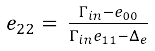Equation 3

Where:

And Transmission Tracking: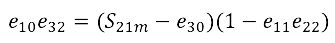Equation 4

The effect of the non-zero delay Thru which is used to connect the ports is not shown here and results in minor modifications of Equation 3 and Equation 4.

Using these error terms, one may now convert raw measurements of S11m, S12m, S21m, and S22m (“m” subscript for raw measured) into actual S11, S12, S21, and S22. The formulas for this calculation are long and can be found in Appendix A.

#### TRL Calibration

The 12-term models in Figure 4 and Figure 5 make physical sense, and the various errors can be ascribed to unique physical features of a vector network analyzer and attached cables. But another error model utilizes Cascade or Transfer parameters. If the actual S-parameters for the DUT are represented by a transfer matrix Sa and matrices X and Y represent the input and output error contributions respectively, then the measurement is: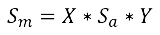Equation 5

Where Sm is the measured Transfer Parameters and Sa is the actual Transfer Matrix of the DUT without errors.

This simple matrix equation may be solved by finding X and Y experimentally and then inverting these matrices to find Sa.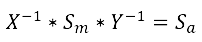Equation 6

This model is beneficial for understanding TRL calibration. Measurement of three calibration standards in the place of Sa above results in twelve independent equations.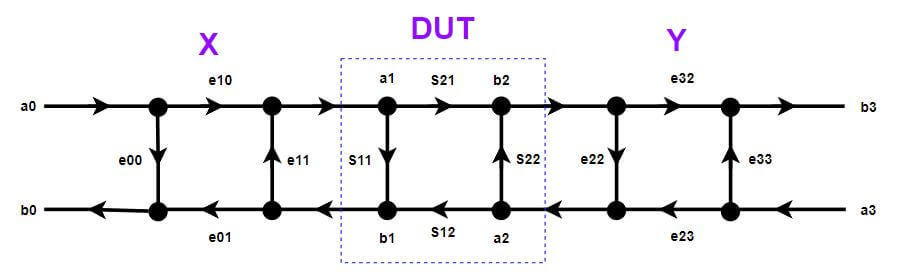Figure 12 – 8 Term Error Network Model

Solving this problem requires the calculation of eight total unknowns in the X and Y matrices, but since S-parameters are ratio-metric, one unknown can be normalized to 1, leaving seven unknowns. With twelve equations and seven unknowns, the problem is overdetermined. With that extra information, the seven values for the X and Y matrices can be calculated along with five other characteristics of the calibration standards which then needn’t be specified. The Thru for TRL is fully specified and usually has zero length. It has four known characteristics so only three more characteristics are needed from the other calibration standards (A non-zero length Thru is technically LRL).

The Line must have an excellent match to the characteristic impedance of the system or its characteristic impedance must be completely known. Perfect knowledge of S12 and S21 of the Line are unnecessary. It provides two more of the needed characteristics.

Finally, the Reflect can be a Short or an Open or anything else on the circumference of the Smith chart. This provides the last of the seven needed characteristics. The same Reflect must be measured by each port of the VNA.

With the X and Y matrices determined, they may be inverted to enable the calculation of actual S-parameters (Sa) from the measured values (Sm).

The full mathematical details of TRL calibration may be found in Appendix B.

#### TRL Can Have Greater Accuracy

The relaxed requirements for the Reflect are very helpful. As previously mentioned, characterization of a Reflect, either Open or Short, is usually given by a delay and a third-order polynomial of the parasitic capacitance of the Open and inductance of the Short; a definition which is constant for every calibration kit of certain manufacture. Clearly, there will be some variation from piece to piece. TRL makes this characterization superfluous and thereby results in greater accuracy. It will be shown that small errors in the estimation of the Open and Short delay values are potentially major contributors to Residual Reflection Tracking and Source Match Errors.

Additionally, in SOLT calibration the uncertainty of the calibration Load mostly determines the Residual Directivity Error which sets the floor for reflection measurements. A good calibration load may have 46 dB Return Loss over a broad band which means the Residual Directivity can be no better than that. Reflection measurements at levels 10 dB higher than this, or -36 dB will have 3.3 dB of uncertainty and approximately 1 dB of uncertainty at -26 dB. On the other hand, a good TRL calibration can result in a Residual Directivity Error as low as -60 dB! This is an astonishing improvement in reflection measurement accuracy.

#### One Path 2-Port Calibration

In this form of calibration, a full 1-port calibration is performed on only one port, not both. This is followed by a Thru normalization. This measurement is performed in only one direction and will deliver S11 and S21 S-parameters, but not S22 or S12 if performed in the forward direction. The 1-port calibration corrects the Source Match, Directivity, and Reflection Tracking Errors. The Thru normalization corrects the Transmission Tracking Error, but the Load Match Error remains uncorrected, so reflection measurements are made against the raw Load Match of the VNA, plus the effect of imperfect cables and connectors attached to the receiving port.

This will cause ripples in low loss S21 measurements such as filter or cable insertion loss where the amount of ripple is highly dependent on the quality of the raw Load Match of the VNA. The TR1300/1 VNA from Copper Mountain Technologies is a one-path 2-port VNA but has a raw Load Match of 28 dB. Because of this, very little error ripple is observed. For a VNA with poor raw Load Match, one could add a good 10 or 15 dB attenuator on the output of the DUT. This will improve the Load Match and reduce ripples in S21 but will also increase trace noise on low-level S21 measurements.

One-path 2-port calibration is recommended when it isn’t possible to perform a full 1-port calibration on the second port. An RF power amplifier might be followed by a large attenuator such that its high-level output doesn’t damage port 2. It isn’t feasible to perform a full 1-port calibration through an attenuator much greater than 15 dB, so full 2-port calibration is impossible. One-path 2-port calibration works well in this case and the attenuator on the output of the amplifier improves the raw Load Match.

#### Response Calibration

In this form of calibration either an Open, a Short, or a Thru with optional Load added are measured. The Open or Short correct for Reflection Tracking only, while the Thru corrects Transmission Tracking only. These are the frequency response errors of the measurement. If a Load is added, then Directivity will be corrected as well. Load Match and Source Match are not corrected. This will generally result in ripples in the measurement and poor overall accuracy.

There may be cases where this method is useful. Where there is a long and lossy cable between the VNA and the DUT and the calibration must be performed on the end of that cable, a full 1-port calibration would be very noisy. It may be better to resort to a response calibration to get any sort of useable measurement at all.

Response calibration can be performed with and without optional Load measurement. The Short or Open alone correct for Reflection Tracking error. Adding Load calibration corrects the Directivity Error as well, but Source Match is not corrected in either case. Figure 13 below shows the difference between these two calibrations for the S11 measurement of a 4 GHz filter.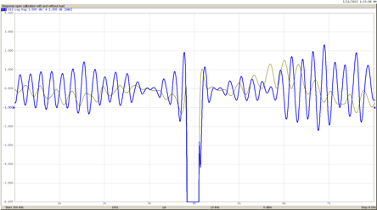Figure 13 – Response Open with (small ripples) and without (larger ripples) optional Load calibration

The large ripples in blue are mostly due to the Directivity error. With Load calibration, the Directivity error is mostly corrected and only the Source Match error remains. Note the faster periodicity of the Directivity error shows the distance traveled to and from the DUT where the incident signal goes out, comes back, and interferes with the leakage signal from the incident signal on the reflection port, effectively two cable lengths. With the Directivity Error mostly removed, the Source Match Error has half this period. The Source Match Reflection occurs directly at the DUT (See e11 in Figure 11). This reflection travels back to the reflection port of the bridge and sees only one cable length. Thus, the phase change vs frequency is half that of the Directivity Error resulting in a slower rate of error ripples.

A Response Thru calibration corrects only Transmission Tracking. It does not correct Source and Load Match. A comparison of Response Calibration vs full 2-port Calibration is shown below in Figure 14. There are significant ripples in the passband measurement of the 4 GHz filter.Figure 14 – Full 2-Port vs Response Thru Calibration

To be very clear, the Response Thru calibration will give a perfectly flat response with the calibration Thru attached. The Transmission Tracking is corrected, and the effects of the Source and Load Match are also corrected while still measuring the calibration Thru. However, if the Thru is replaced by a short length of cable, there will now be a phase delay and the Source and Load Match Errors will phase in and out, creating ripples as seen below in Figure 15. For comparison, a measurement using 2-Port One Path calibration is included which only has the Load Match Error.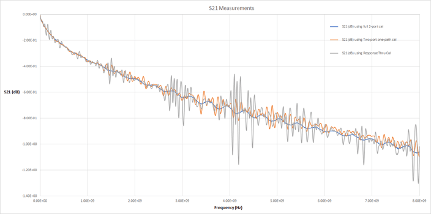Figure 15 – 2 ft cable measurement, full 2-port, 2-Port One path and Thru response calibrations

In Figure 15 one can see the small ripples in the blue full 2-Port calibration measurement due to Residual Directivity error. The larger ripples in the orange 2-Port One Path calibration measurement adds the raw Load Match error to the Residual Directivity and finally the largest ripples in the grey Response Thru calibration measurement shows the effects of raw Directivity plus raw Source Match and raw Load Match errors. Here, the Thru calibration only corrects the frequency response, or the basic slope of the curve.

#### Residual Errors

As mentioned previously, residual errors are the remaining errors after calibration has been performed. For example, raw Directivity can be evaluated by placing a perfect Load on the port of the VNA and measuring the leakage signal from the incident signal out of the reflection port of the bridge which should ideally have no signal present at all. If this signal is recorded, it can be subtracted from the reflection port signal leaving only the true reflection signal. Unfortunately, a perfect Load does not exist and any reflection from the imperfect Load will be present on the reflection port, thus setting the floor for the lowest possible reflection measurement. This will be about 37 dB for an economy-grade calibration Load and perhaps 43 dB for a higher precision Load. These values set the Residual Directivity.

An ACM with its databased load can achieve 47 dB of Residual Directivity. The effectiveness of the calibration and the magnitude of the residual errors depend greatly on the accuracy of the calibration standards used for calibration.

The various residual errors may be estimated as follows: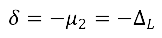Equation 7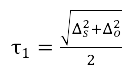Equation 8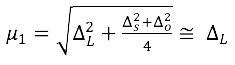Equation 9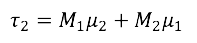Equation 10

Where:

δ is the Residual Directivity, τ1 is Residual Reflection Tracking, µ1 is Residual Source Match, and τ2 is Residual Transmission Tracking.

ΔL is the error, or Return Loss, of the Load calibration standard.

Δs and Δo are the errors of the Short and Open calibration standards.

µ2 is the Residual Load Match

M1 and M2 are the raw, uncorrected Source and Load Match values

For the Residual Source Match term µ1, the Short and Open errors are small compared to the Load error so µ1 is approximately equal to the Load error ΔL.

It is also worth noting that the Open and Short calibration standard errors can be set to zero for TRL calibration as the exact value of the Reflect used is unimportant for this method. This tells us that the Residual Reflection Tracking Error will be very small indeed. Still, not quite zero since these equations are a simplified version of much longer equations. The simplified versions contain only dominant terms.

#### How Do the Residual Errors Effect the Measurements?

Now that the Residual Errors are understood, it is possible to use them to determine the uncertainty of an S-parameter measurement. The uncertainty of a 1-port measurement is as follows: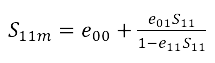Equation 11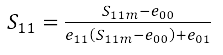Equation 12

Note how the measured value of S11 approaches e00, the Residual Directivity, as the actual value of S11 goes to zero. We also know from before that e00 is predominantly determined by the uncertainty, or Return Loss, of the calibration Load. This equation applies for a 1-port measurement of a DUT, or one loaded with a perfect 50 Ohms and not terminated by the other port of the VNA.

If the other side of the DUT is terminated by the second port of the VNA, then all S-parameters and all residual terms in both directions must be in the equation: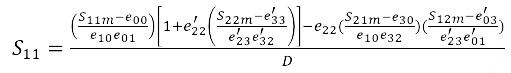Equation 13

Where: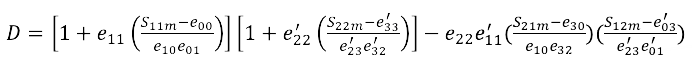The primed terms are residual errors from the reverse measurements and come from the reverse 12-Term model in Figure 5.

This equation is much less instructive. The full equations for the other three S-parameters are similar and are shown in Appendix C.

#### Reflection Measurement Uncertainty

The following simplifications on the effect of uncertainties for reflection and transmission are proposed in the EURAMET cg-12 document in “Guidelines on the evaluation of vector network analyzers”.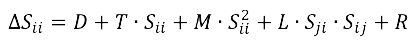Equation 14

Where (all in linear terms):

ΔSii is a 1-port reflection measurement uncertainty

Sii is the actual reflection measurement

Sji and Sij are forward and reverse transmission measurements

D is the Residual Directivity

T is the overall effect of reflection tracking and non-linearity

M is the residual Source Match

L is the residual Load Match

And R represents the effects of random contributions such as thermal drift, cable flexure, connector repeatability, trace noise, and ambient conditions.

If the measurement is a true 1-port measurement and not terminated by the second port of the VNA, then the term with Load Match would be zero.

In practice, the Residual Directivity dominates at low reflection levels (left-hand side) and Residual Reflection Tracking dominates the error at high reflection levels (right-hand side of the chart below). This curve for the S5065 VNA is shown in Figure 13.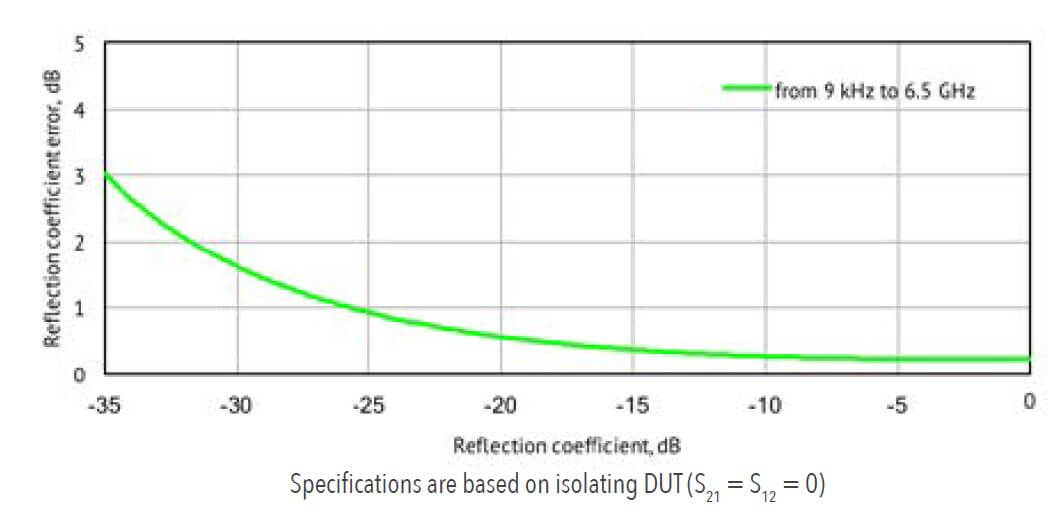Figure 16 – Reflection Accuracy, S5065 VNA

Some conclusions can be drawn from this formula.

• Residual Directivity Error dominates for low reflection measurements
• Residual Directivity is greatly dependent on the calibration Load uncertainty. For the least uncertainty, a precision calibration Load should be used.
• Residual Reflection Tracking and Source Match dominate for high reflection measurements
• Source Match Error falls off quickly due to the squared Sii term
• Load Match Errors have some effect for low insertion loss measurements but fall off quickly due to the essentially squared term, Sij*Sji. Adding a good 6 to 10 dB attenuator to the test cable at the output of the DUT and calibrating it out will improve the Residual Load Match and will reduce the effect of the term containing “L”.
• Random errors such as cable flexure and ambient temperature changes should be controlled as much as possible

#### Transmission Measurement Uncertainty

The simplified uncertainty for Transmission measurements as proposed by EURAMET is: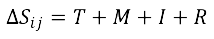Equation 15

Where T is the overall effect of tracking and non-linearity in dB

M is the error term due to mismatch in dB

I is the error term due to isolation (cross-talk) in dB

R represents all random contributions in dB

In practice, the effect of linearity error occurs at low insertion loss measurements with source power set to high values. This effect tapers off quickly for higher insertion loss as the power into the receiver decreases. Transmission Tracking Error then takes over until at low levels, Trace Noise dominates as the signal level approaches the noise floor. This bathtub-shaped curve is shown in Figure 14.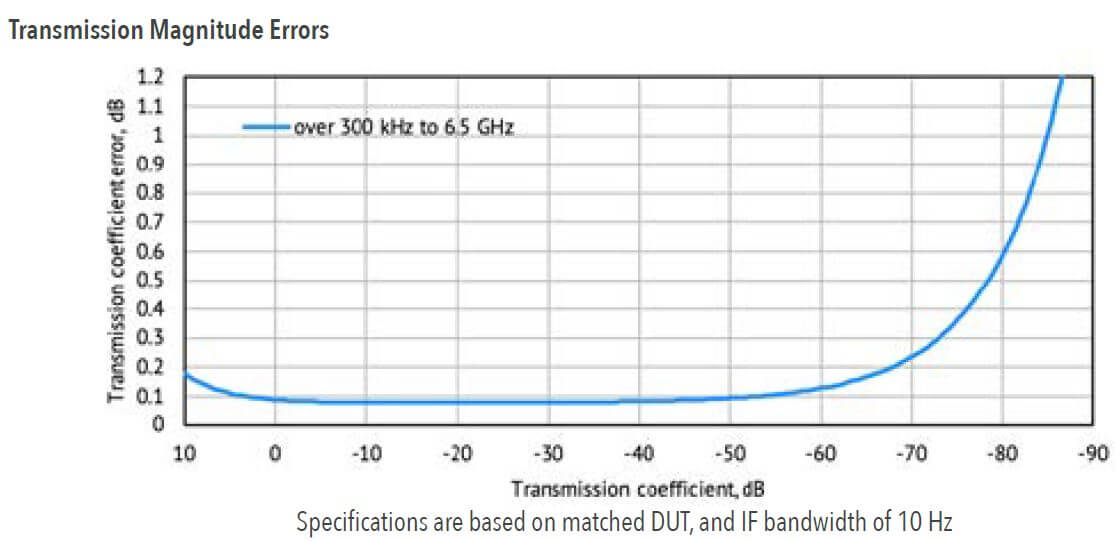Figure 17 – Transmission Accuracy, S5065 VNA

The Residual Transmission tracking is defined by Equation 10, the sum of the products of raw Source Match and Residual Load Match and Residual Source Match and raw Load Match. The two residual terms are approximately equal to the calibration Load uncertainty as long as the Open and Short calibration standard uncertainties are reasonable. If either of these two standards are poorly defined, (particularly the delay specification) then Residual Source Match would be compromised, and Residual Transmission Tracking would suffer.

This section is meant to be an introduction to Residual Errors and uncertainties. For an in-depth analysis of the uncertainties of a particular VNA measurement system one could obtain the Insight software from Maury Microwave. It is well documented and quite powerful. Alternatively, METAS VNA Tools can also be used for VNA uncertainty analysis.  METAS is the Federal Institute of Metrology in Switzerland.

Some conclusions can be drawn from the uncertainty formula:

• Residual Transmission Tracking dominates the measurement uncertainty over a large range
• Residual Transmission Tracking error is greatly dependent on the calibration Load uncertainty and to a lesser extent, the uncertainties of the Open and Short. For least uncertainty, a precision calibration Load should be used.
• Avoid non-linearity errors by performing measurements with receiver power below 0 dBm
• Random errors such as cable flexure and ambient temperature changes should be controlled as much as possible

#### A Practical Example

For example, if a 60 dB attenuator is to be measured with a Copper Mountain Technologies S5065 in a 10 Hz IF Bandwidth with source power of 5 dBm, we can calculate the uncertainty of the measurement. The mean noise floor of the S5065 is -130 dBm/Hz. The 10 Hz noise floor is -120 dBm but we need to find the 3σ maximum contribution of this noise. To do this, we turn to Raleigh statistics.

The Raleigh distribution of a complex vector with zero center and sigma 0.1 on both real and imaginary components is shown in Figure 15.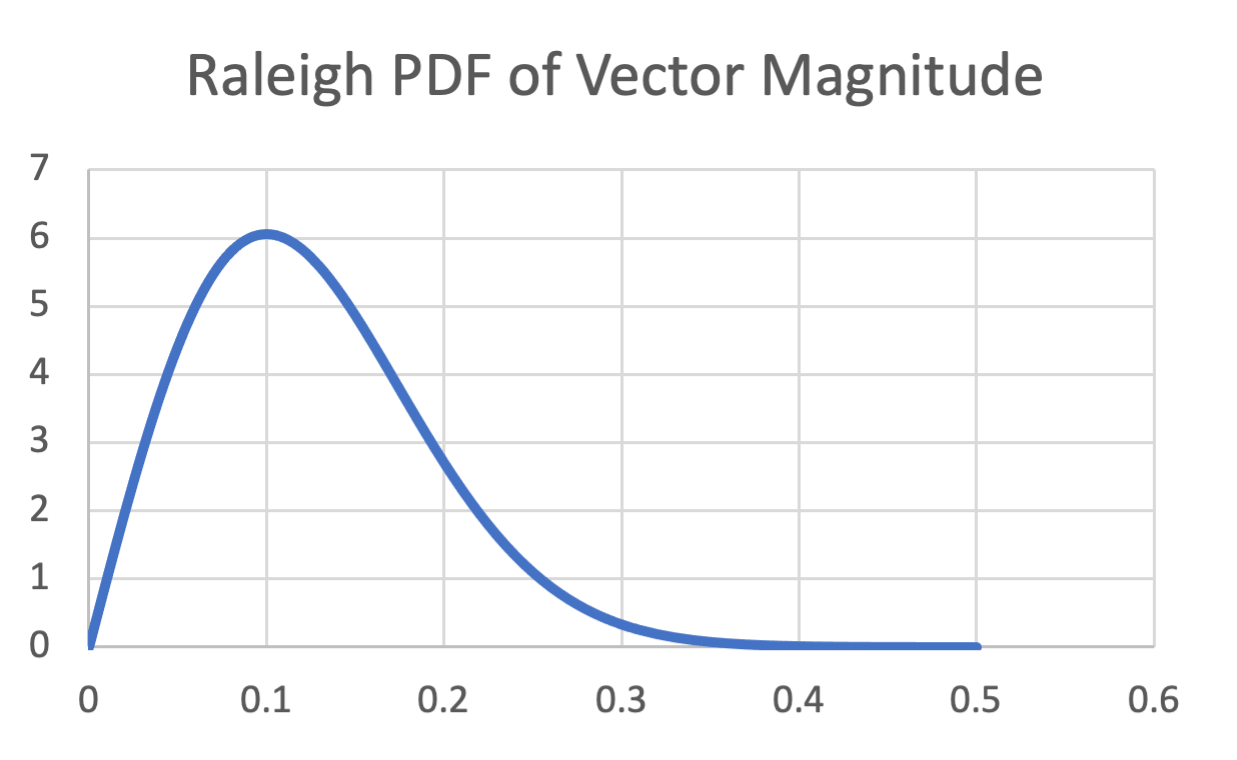Figure 18 – Raleigh Probability Distribution

Notice that the distribution is always positive valued as the magnitude of a vector may be taken to always be positive. This differentiates it from a Gaussian probability distribution.

The mean of a Raleigh Distribution is: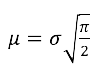Equation 16

Where σ is the standard deviation of the real and imaginary components of the complex value.

The standard deviation of a Raleigh Probability Distribution is: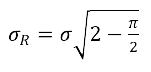Equation 17

Therefore, if we know the mean noise level µ, and want a 3σ maximum deviation (99% confidence interval) then that ratio is: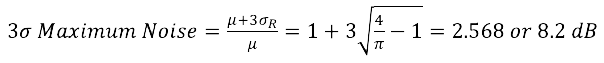Equation 18

The 8.2 dB is rounded up to 10 dB to account for the small contribution of other sources of random noise.

With a 10 Hz noise floor of -120 dBm we add the 10 dB to get the Maximum 3σ vector noise contribution and have -110 dBm. Subtracting the source power of 5 dBm and we have a -115 dB signal to maximum noise ratio. The expected uncertainty of the 60 dB attenuator measurement due to receiver thermal noise alone is then:Equation 19

For a measurement of an 80 dB attenuator, this rises to 0.16 dB and of course the uncertainty goes to infinity at 110 dB. This is the major contributor to the curve on the right side of Figure 14.

A full accounting of Transmission Uncertainty must include all factors though. If we assume a matched load, we can ignore the “M” term in Equation 15. All terms for the 60 dB attenuator measurement are then: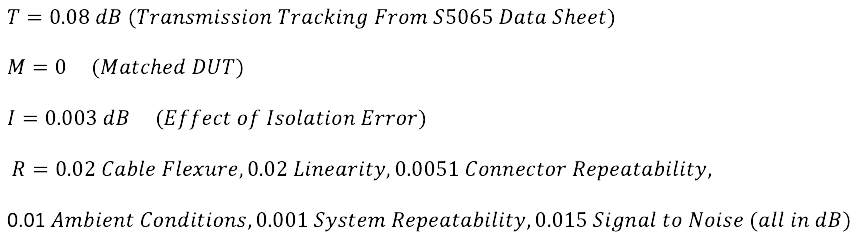Weighting factors of 0.5 are used for Gaussian distributed errors and 0.578 for the Rectangular Isolation and Ambient errors. Finally, for a 1.96σ expectation (95% Confidence), the weighted sum is multiplied by 1.96: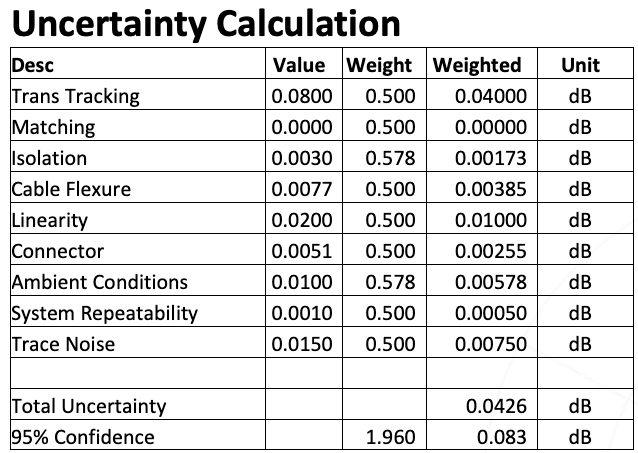Table 1 – Uncertainty Calculation

Calculated total uncertainty for the Transmission measurement, the square root of the sum of the squares of the weighted uncertainties of the 60 dB attenuator is ± 0.08 dB with 95% confidence and the datasheet specification is ±0.2 dB for this VNA model.

#### Phase Uncertainty

The uncertainty of the phase is determined directly from the uncertainty of the magnitude. Taking the magnitude uncertainty to be a small vector added to the measured value, the phase uncertainty may be estimated by assuming a worst-case condition where the uncertainty is at right angles to the measurement. Taking the arcsine of the ratio gives the maximum possible angle of the uncertainty.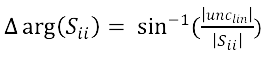in radians, uncertainty, and Sii in linear format
Equation 20in radians, uncertainty in dBs
Equation 21

For the ± 0.14 dB on the Transmission Error from the last section the phase error would be ± 0.92 degrees.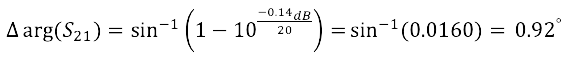### Random Errors in VNA Measurement

#### Trace Noise

The trace noise of a transmission measurement has two contributors. One is simply due to the signal to noise ratio against the receiver noise floor. This is dominant for low-level measurements where the total noise is the specified noise floor plus 10 dB and the trace noise contribution is found using Equation 19. At higher levels, the contribution of the receiver noise floor falls off rapidly and the remaining noise can be attributed to Stimulus and Local Oscillator (LO) phase noise. The resulting noise power of the phase noise of the IF signal results in measurement fluctuations. Lowering the IF Bandwidth of the measurement will reduce the noise for low-level measurements but might have little effect for high-level measurements dominated by phase noise. Phase noise is not flat and increases with lower frequency offset from the IF carrier frequency. Therefore, the noise difference between a 10 Hz and 100 Hz IF bandwidth measurement will not be a factor of ten at high levels as it is at lower measurement levels where the noise is dominated by statistically flat, white noise.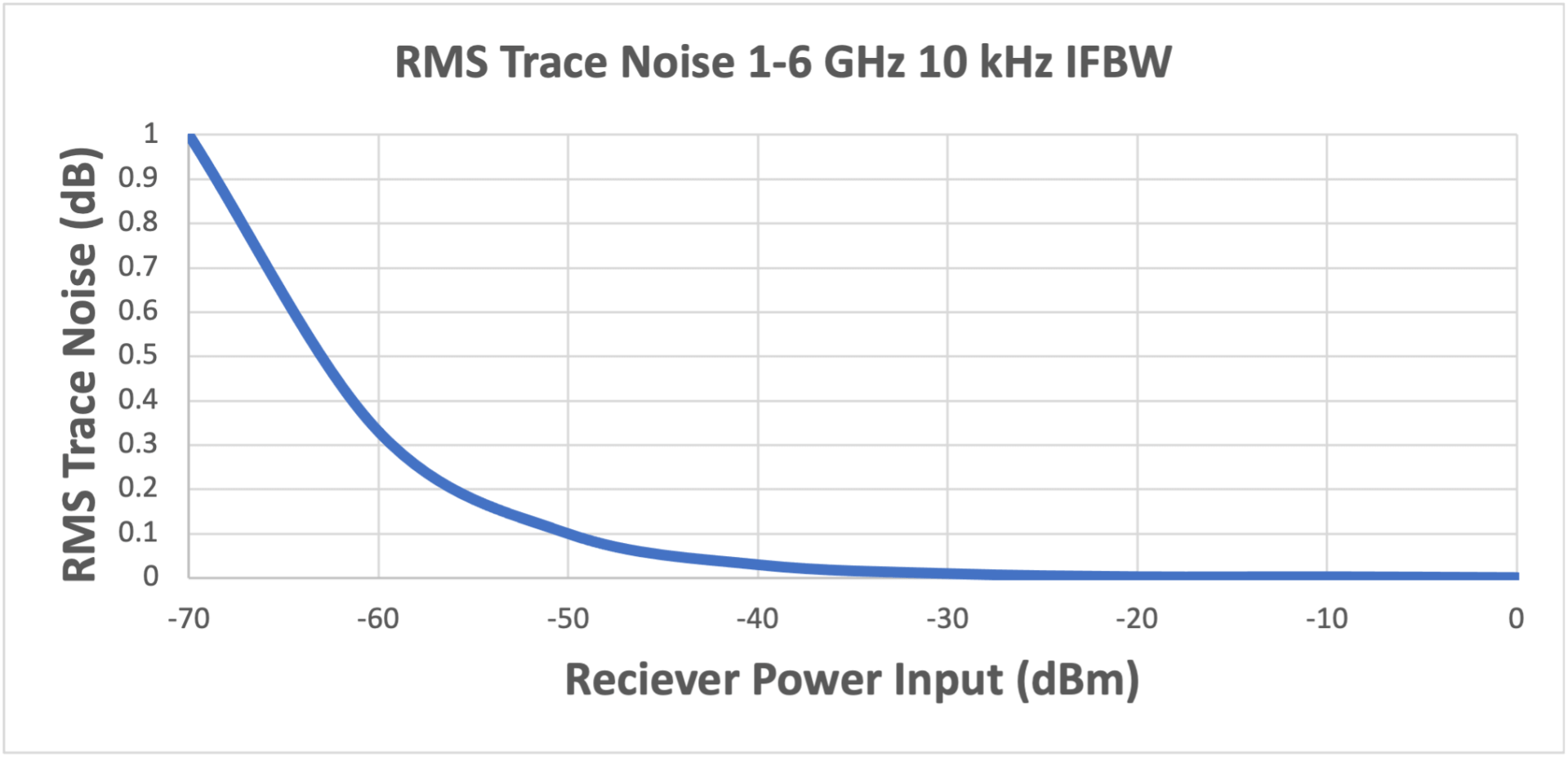Equation 22 – Trace Noise vs Received Power

#### Thermal Effects

It is natural for test equipment to have a certain amount of thermal drift and a VNA is no exception. Specified measurement drift at high levels of reflection or transmission is 0.02 dB/degree C. It is possible to recalibrate a VNA after a temperature change, but time must be allowed for the internal temperature to stabilize. Figure 19 shows the internal temperature of a pair of TR1300/1 VNAs after power-up and insertion into a 45-degree C temperature chamber.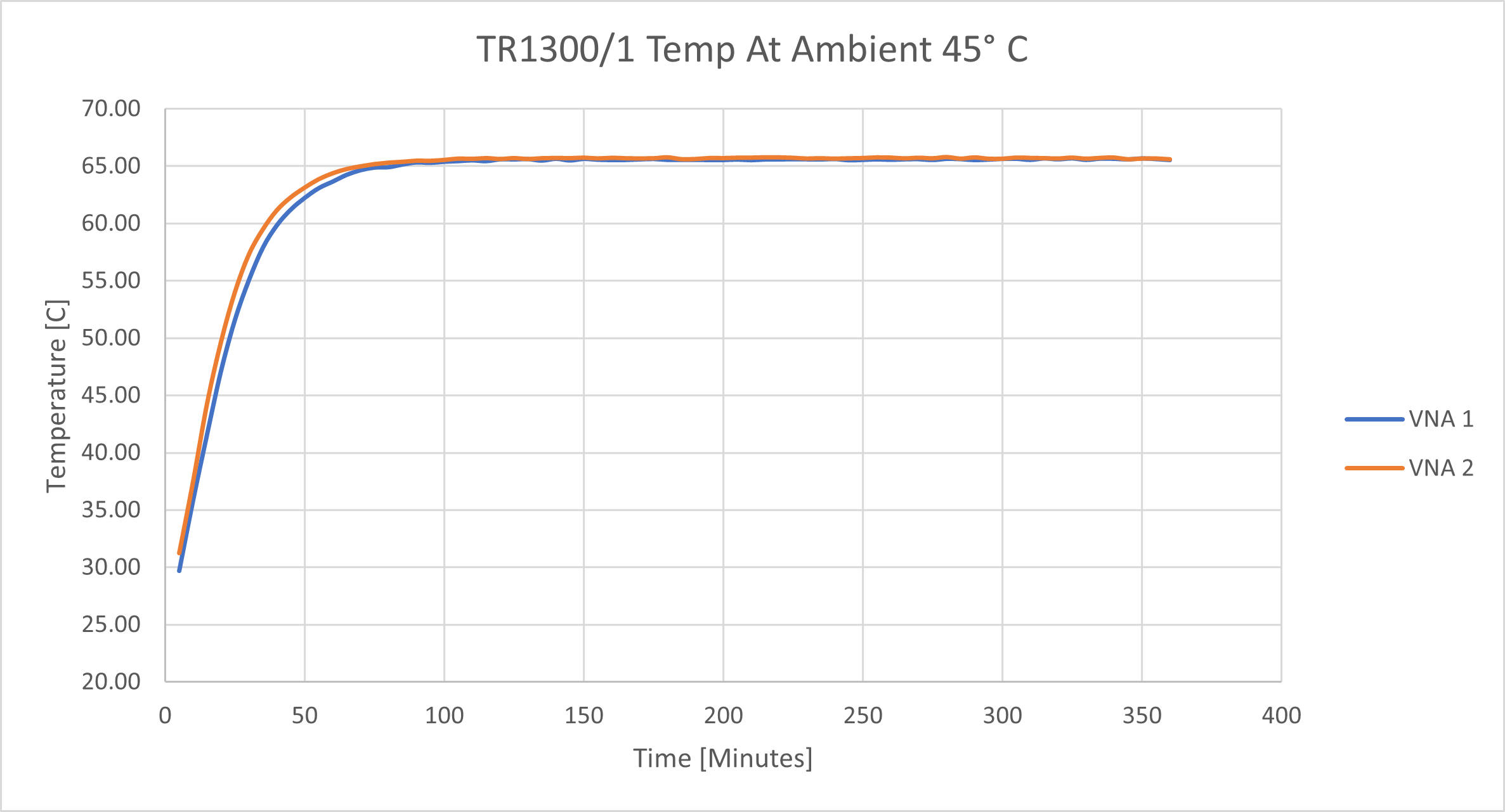Figure 19 – TR1300/1 Temperature Rise and Stabilization

An hour is required for the VNA internal temperature to stabilize at about 20°C above the ambient temperature. For best measurement accuracy, allow a VNA to warm up for one hour before performing calibration.

#### Mechanical Effects

It cannot be overstressed how important it is to minimize cable movement after VNA calibration has been performed. Small amounts of flexure will cause changes in the delay or phase shift through a test cable which puts the vector error correction out of phase with the errors it should correct. Ripples in the measurement will result. A cable will have a natural bend, and it is important to align the cable such that the connection to the DUT follows this curvature. Flexing a cable against its natural bend will cause excess phase changes and instability.

Many cables have a substantial change in properties over temperature. PTFE has a molecular state transition near 25°C which causes a jump in the phase velocity. Maintaining a constant temperature in the lab will help prevent this and reduce thermal drift within the VNA as well.

It is important to check the stability of the cables before a measurement is performed. This may be done by attaching a calibration Load to the end of the cable and measuring S11 while moving the cable about. It is particularly important to check the cable near the connectors as this flexure point is where cables fail. If there is any significant movement of this Return Loss measurement during cable movement, the cable should be discarded. Cutting it in half before discarding it will prevent another engineer from trying to salvage it and perhaps making a lengthy series of useless measurements. In a production environment, this test should be performed at the beginning of every shift.

Connectors have an uncertainty/repeatability of perhaps 0.0051 dB. Cables must be attached and unattached in the process of calibration and re-connection to the DUT of course. It is important to inspect the connectors and ensure the surfaces are free of contaminants. Contaminated connectors will have a much higher uncertainty/repeatability. A connector may be cleaned by twirling raw cotton on to the end of a toothpick and wetting it with alcohol. Store-bought cotton swabs contain a glue which holds the cotton to the stick which will dissolve in alcohol and contaminate the connector.

### Conclusion

We defined the science of Metrology and attempted to show how this science applies to VNA measurements. We have examined the various methods of calibration for a VNA which correct all or only some systematic errors. The effects of leaving one or more errors uncorrected were shown. Residual errors, those errors which remain after calibration were defined, and accepted methods for determining measurement uncertainty from them were demonstrated. The relationship between expected residual errors and the uncertainties (or quality) of the calibration kit employed were emphasized.

Errors caused by random factors such as cable movement and ambient conditions were discussed, and an awareness of these errors and their effects should intuitively lead to more meticulous measurement methodology to minimize them.

### Appendix A:   Full 2-Port Calibration error correction

Corrected S-parameters S11, S12, S22 and S21 may be calculated from raw measured S11m, S12m, S22m and S21m, and error terms e00, e01, e10, e11, e22 and e32 from the forward 12-Term model of Figure 4 and e01’, e11’, e22’, e23’, e32’ and e33’ from the reverse model of Figure 5. These errors are determined through the process of calibration.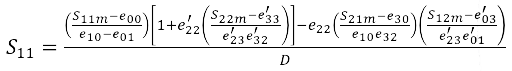Equation 23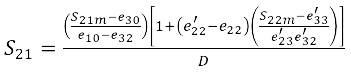Equation 24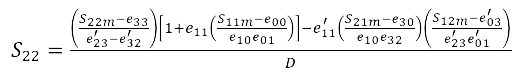Equation 25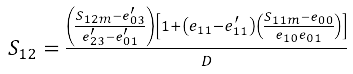Equation 26

Where: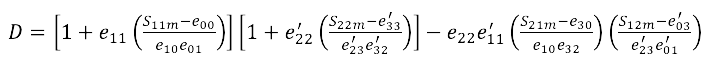### Appendix B: TRL Correction

Corrected S-parameters S11, S12, S22 and S21 may be calculated from raw measured S11m, S12m, S22m and S21m, and error terms e00, e11, e01, e10 from the left-hand error box and e22, e32, e33 and e23 from the right-hand error box from the 8-Term error model of Figure 12.

If the left-hand S-parameter error box is: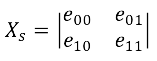Equation 27

And the right-hand side S-parameter error box is: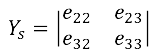Equation 28

Then these two S-parameter matrices may be converted to Transfer matrices: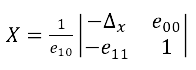Equation 29

Where the determinant: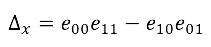And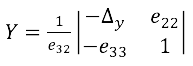Equation 30

Where the determinant: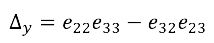The Transfer parameter measurement, Tm, with errors is then: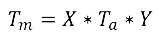Equation 31

Where Ta is the actual Transfer Parameter matrix (corrected).

The actual Transfer parameter matrix, Ta, may be found with simple matrix math: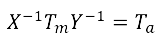Equation 32

Expanding Equation 31: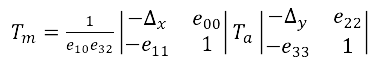Equation 33

We’ll soon need the determinant of Ta, where: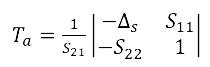Equation 34

Which is: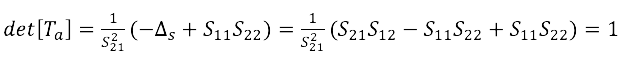Equation 35

If we let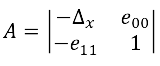and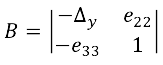from Equation 33 we can re-write it as: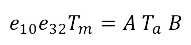Equation 36

And taking the determinant of both sides: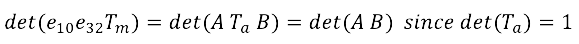Equation 37

Then: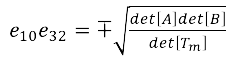Equation 38

Where: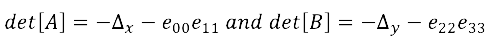and from before: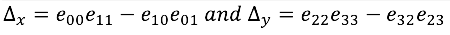The 1-port calibrations of ports 1 and 2 provided terms Δx, e00, e11 and product e01e10 from port 1 and Δy, e22, e33 and product e23e32 from port 2. We only needed to find the transmission terms e10e32 which we now have from Equation 39. Now we invert A and B: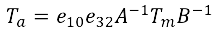Equation 39

And finally: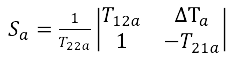Equation 40

Note the ambiguity of the sign of e10e32 in Equation 39. This amounts to a 180-degree phase shift in the transmission error terms. It is necessary to apply some common sense to the calculation in order to choose the correct sign.